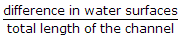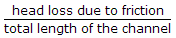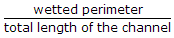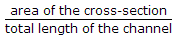# Civil Engineering - Hydraulics

Exercise : Hydraulics - Section 2
26.
A steady uniform flow is through
a long pipe at decreasing rate
a long pipe at constant rate
an expanding tube at constant rate
an expanding tube at increasing rate
a long pipe at increasing rate.
Explanation:
No answer description is available. Let's discuss.

27.
Cavitation is caused by
Low pressure
High pressure
Low velocity
High velocity
None of these.
Explanation:
No answer description is available. Let's discuss.

28.
Mach number is the ratio of inertia force to
viscosity
surface tension
gravitational force
elasticity.
Explanation:
No answer description is available. Let's discuss.

29.
Hydraulic gradient is equal to difference in water surfaces